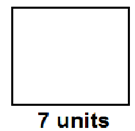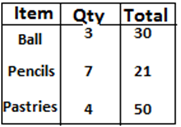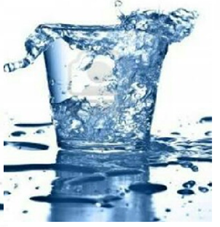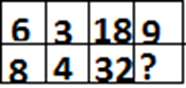# CREST Mathematics Olympiad Class 3 Sample Papers

## Syllabus:

Section 1: Numerals, Number Names and Number Sense (4-digit numbers), Computation Operations, Fractions, Length, Weight, Capacity, Temperature, Time, Money, Geometry, Data Handling.

Achievers Section: Higher Order Thinking Questions - Syllabus as per Section 1

 Q.1 Solve the equation. 0 × 4 × 9 =
 Q.2 There were 49 students in a class. 1/7th of the class were girls. How many boys were there in the class?
 Q.3 What is the sum of lengths of other three sides?Q.4 With reference to the table in image, what is the sum of the prices of one ball and a pencil?Q.5 The capacity of the glass is 250 ml. However Dipika unknowingly kept pouring water from a jug with capacity 750ml which was now empty. How much ml of water do you think has spilled?Q.6 3 score eggs were purchased by Ramesh of which 12 broke while returning home. How many eggs did Ramesh finally bring home?
 Q.7 23 minutes = ___ hours.
 Q.8 The face value of 6 in 6132 is:
 Q.9 How much do 12 Rs. 20 notes, 7 Rs.5 coins and 100 Rs.100 notes amount to?
 Q.10 Which number will replace '?' in the given table?Sample PDF of CREST Mathematics Olympiad for Class 3: